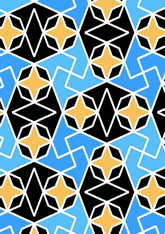# Mir Chaqmaq mosque, Yazddata180/IRA2004

The coloured interlacing is omitted here. An alternative encoding is to be found in Mir Chaqmaq mosque, Yazd.

## Geometry

• The symmetry group of the tiling is 4*2 (p4g).
• All the internal angles of the constituent polygons are a multiple of 45°.
• Contains two regular two-pointed star polygons with vertex angle of 45°.
• Contains one regular four-pointed star polygon with vertex angle of 45°.
• There is one non-regular reflective tile and one reflective pair.
• The tiling satisfies the two-colour condition.
• The tiling is edge-to-edge.
• As drawn, contains about 205 polygons.

## References

Publications referenced: